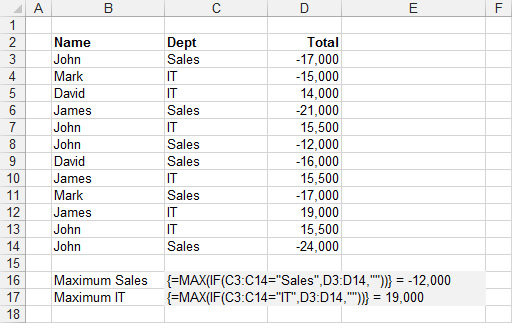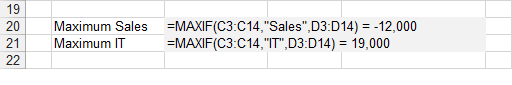MAXIF

Returns the maximum value in a range of values that match a certain condition.

'rgeCriteria - The range of cells containing the criteria you want to check.
'sCriteria - The criteria value you want to match.
'rgeMaxRange - The range of corresponding values you want the maximum of.

Public Function MAXIF(ByVal rgeCriteria As Range, _
ByVal sCriteria As String, _
ByVal rgeMaxRange As Range) As Single

Dim iconditioncolno As Integer
Dim inumberscolno As Integer
Dim lrowno As Long
Dim sngmax As Single
Dim vcellvalue As Variant

iconditioncolno = rgeCriteria.Column
inumberscolno = rgeMaxRange.Column
For lrowno = 1 To rgeCriteria.Rows.Count
vcellvalue = rgeCriteria.Parent.Cells(rgeCriteria.Row + lrowno - 1, inumberscolno).Value
If rgeCriteria.Parent.Cells(rgeCriteria.Row + lrowno - 1, iconditioncolno).Value = sCriteria And _
IsNumeric(vcellvalue) = True Then
If sngmax = 0 Then sngmax = vcellvalue
If vcellvalue > sngmax Then sngmax = vcellvalue
End If

If sngmax <> 0 And IsEmpty(vcellvalue) = True Then Exit For
Next lrowno

For lrowno = 1 To rgeCriteria.Rows.Count
vcellvalue = rgeCriteria.Parent.Cells(rgeCriteria.Row + lrowno - 1, inumberscolno).Value
If rgeCriteria.Parent.Cells(rgeCriteria.Row + lrowno - 1, iconditioncolno).Value = sCriteria And _
IsNumeric(vcellvalue) = True Then
If rgeCriteria.Parent.Cells(rgeCriteria.Row + lrowno - 1, inumberscolno).Value > sngmax Then
sngmax = rgeCriteria.Parent.Cells(rgeCriteria.Row + lrowno - 1, inumberscolno).Value
End If
End If

If sngmax <> 0 And IsEmpty(vcellvalue) = True Then Exit For
Next lrowno

MAXIF = sngmax
End Function

MAXIFS was added as a built-in function in 2016 Jan.
There is an Array Formula equivalent if you do not want to use the user defined function (see below).
This includes hidden rows.
This user defined function is similar to the built-in SUMIF function.Array Formula

To find out more about Array Formulas please refer to the Array Formulas section.
To find out more about the individual functions please refer to the MAX function and IF function pages.
This example illustrates how to use an array formula to obtain the maximum value in a column based on a condition.The formula in row 16 returns the largest total in the "Sales" department.
The formula in row 17 returns the largest total in the "IT" department.
The formula [C3:C14="Sales"] returns an array of 1's and 0's indicating if the value in column "C" satisfies the condition.
Every number 1 in this array represents the value True in the IF statement and therefore has its corresponding value returned from column "D".
Every number 0 in this array represents the value False in the IF statement and therefore an empty string is returned in this case. The MAX function will ignore any string values.
The use of the empty string is to ensure that the formula works with both positive and negative numbers.
This array of numbers is then passed to the MAX() function to obtain the largest number.
Remember that you must press (Ctrl + Shift + Enter) to enter the formulas.

Important

You cannot use column references (such as "A:A" or "D:D") in your array formulas.
Any cell ranges passed to an array formula must have the same number of rows. This is to ensure that all the temporary arrays used are the same length.

© 2019 Better Solutions Limited